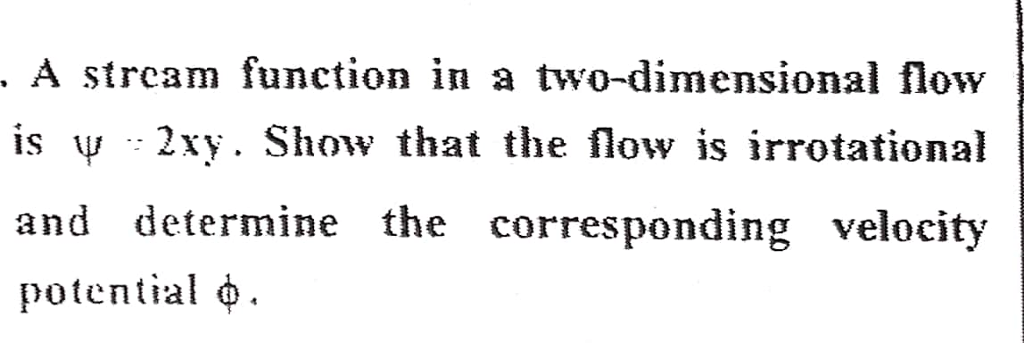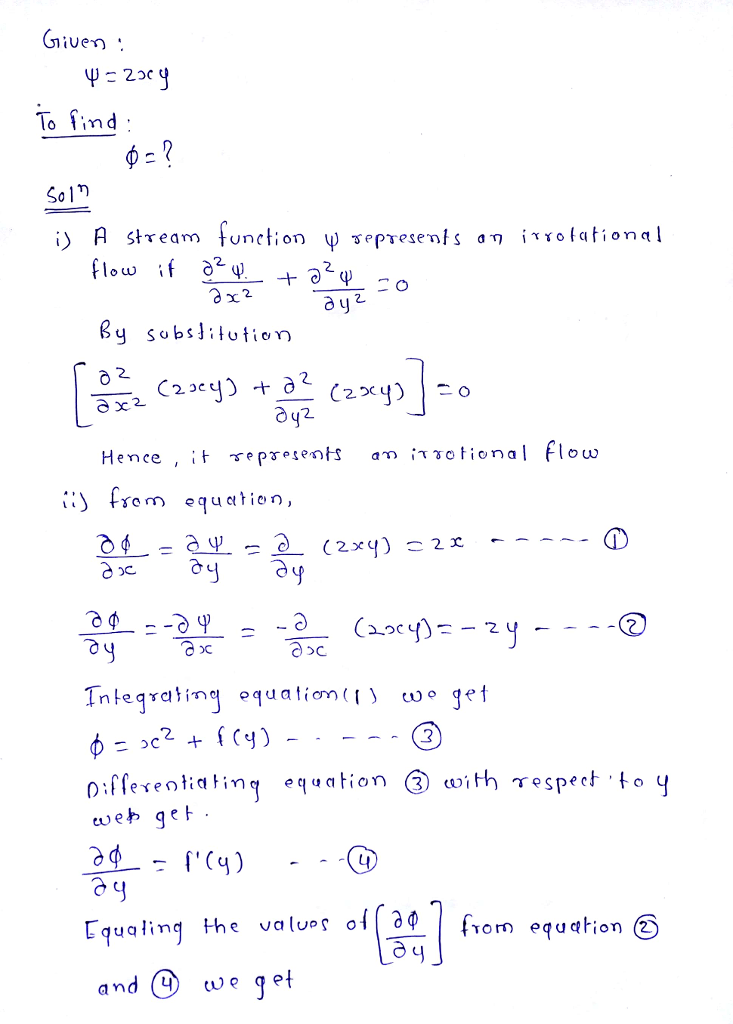# A stream function in a two-dimensional flow is ψ = 2xy. Show that the flow is irrotational (potential) and determine the corresponding velocityp otential function φ.

Question-AnswerCategory: Fluid MechanicsA stream function in a two-dimensional flow is ψ = 2xy. Show that the flow is irrotational (potential) and determine the corresponding velocityp otential function φ.

A stream function in a two-dimensional flow is ψ = 2xy. Show that the flow is irrotational (potential) and determine the corresponding velocityp otential function φ.